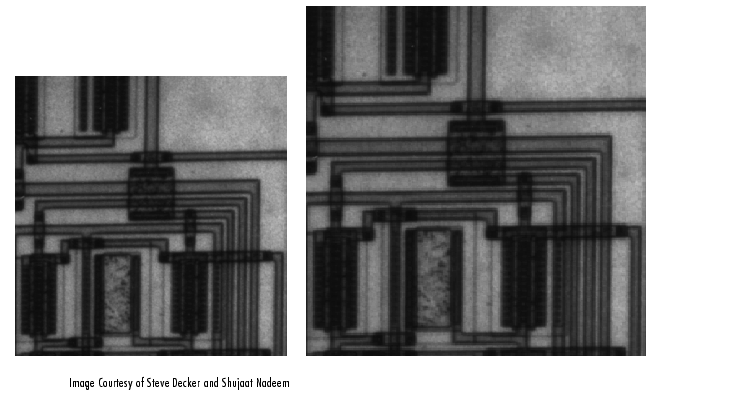Image Processing Toolbox User's GuideResizing an Image

To change the size of an image, use the `imresize` function. Using `imresize`, you can

Specifying the Size of the Output Image

Using `imresize`, you can specify the size of the output image in two ways:

Using the Magnification Factor

To enlarge an image, specify a magnification factor greater than 1. To reduce an image, specify a magnification factor between 0 and 1. For example, the command below increases the size of an image by 1.25 times.

• ```I = imread('circuit.tif');
J = imresize(I,1.25);
imshow(I)
figure, imshow(J)```

Specifying the Size of the Output Image

You can specify the size of the output image by passing a vector that contains the number of rows and columns in the output image. If the specified size does not produce the same aspect ratio as the input image, the output image will be distorted.

The following command creates an output image with 100 rows and 150 columns.

• ```I = imread('circuit.tif');
J = imresize(I,[100 150]);
imshow(I)
figure, imshow(J)
```

Specifying the Interpolation Method

By default, `imresize` uses nearest-neighbor interpolation to determine the values of pixels in the output image, but you can specify other interpolation methods. This table lists the supported interpolation methods in order of complexity. See Interpolation for more information about these methods.

 Argument Value Interpolation Method `'nearest'` Nearest-neighbor interpolation (the default) `'bilinear'` Bilinear interpolation `'bicubic'` Bicubic interpolation

In this example, `imresize` uses the bilinear interpolation method.

• ```Y = imresize(X,[100 150],'bilinear')
```

Using Filters to Prevent Aliasing

When you reduce the size of an image, you lose some of the original pixels because there are fewer pixels in the output image. Aliasing that occurs as a result of size reduction normally appears as "stair-step" patterns (especially in high-contrast images), or as moiré (ripple-effect) patterns in the output image.

When you specify either bilinear or bicubic as the interpolation method, `imresize` automatically applies a lowpass filter to the image before interpolation to limit the impact of aliasing on the output image.

 Note    Even with lowpass filtering, resizing an image can introduce artifacts, because information is always lost when you reduce the size of an image.

The `imresize` function does not apply a lowpass filter if nearest-neighbor interpolation is used. Nearest-neighbor interpolation is primarily used for indexed images, and lowpass filtering is not appropriate for these images.

When using `imresize` to reduce the size of an image, you can specify the size of the lowpass filter or specify a filter of your own creation. For example, the following code specifies a 9-by-9 filter. (The default size is 11-by-11.) If you specify the value `0` (zero), `imresize` does not perform lowpass filtering.

• ```J = imresize(I,'bilinear',9);
```

For more information about specifying a filter, see the reference page for `imresize`.Interpolation Rotating an Image